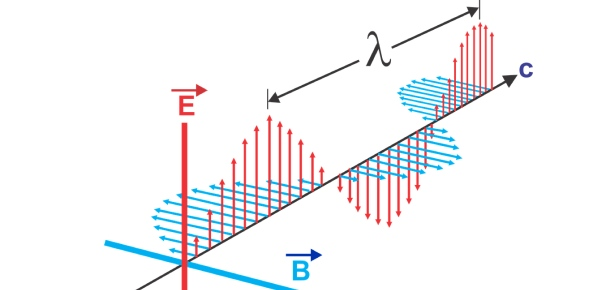Electromagnetic Wave Quiz

6 QuestionsSettingsCan you answer the following questions on the electromagnetic spectrum? Test your knowledge on this physics quiz to see how you do and compare your score to others.

• 1.
What are the properties of  electromagnetic waves?
• A.

Frequency

• B.

Wavelength

• C.

None of the above

• D.

A and B

• 2.
A ________ can travel through empty space and is produced by particles in motion
• A.

Wave

• B.

Electromagnetic wave

• C.

• D.

None of the above

• 3.
• A.

• B.

• C.

Gamma Rays

• D.

All of the above

• 4.
Which electromagnetic wave can you see with the naked eye?
• A.

Infrared Waves

• B.

Carrier waves

• C.

• D.

Visible Light

• 5.
Which animal can detect infared waves?
• A.

Moccasins

• B.

Water moccasins

• C.

Penguins

• D.

Polar bears

• 6.
Does a radio antenna convert electricalmagnetic energy radiation to electrical energy?
• A.

True

• B.

False

Related Topics